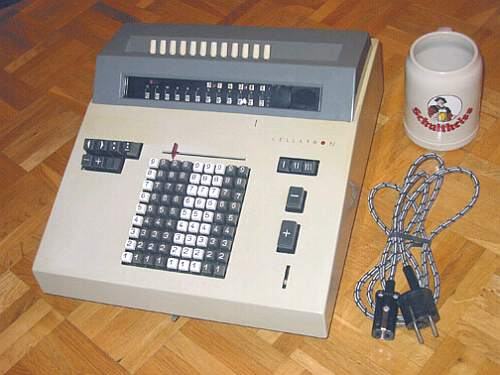previous <<==>> next

CELLATRON  R 31Have a look inside - and ...
To show & demonstrate the BEAUTY OF THE HARDWARE ...
... Have a look at the Design Procedure for making an ACRYLIC COVER ...
 ``` TECHNICAL DATA of the Electro-Mechanical Calculator CELLATRON R 31 ************************************************************************ A decendent of the MERCEDES-EUKLID, Invented by CHRISTEL HAMANN in 1906 Serial-Number: 217229 (inside: 25944 = Chassis; 23821 = Carriage) Dimensions: (ca.) Width = 13_1/2" / 34 cm Depth = 15_1/2" / 39 cm Height = 7_1/2" / 19 cm Weight: (ca.) 33 lbs / 15 kg Power Supply: 220 V / 200 W (grounded, AC ) Mechanics: 9*9 Full-Keyboard Proportional-Lever / Proportional-Hebel Functions: Add, Subtract, Multiply, Divide Division-Automatic! Registers: I Counter = 6 Decimals II Arithmetic = 12 Decimals III Input = 9 Decimals Manufacturer: VEB Kombinat Rechentechnik ( Successor of MERCEDES BUEROMASCHINEN WERKE Berlin ) Zella-Mehlis in Thueringen ( GDR ) Germany 1967 BASICS & HOW TO USE the CELLATRON R 31 ****************************************** (A) Clearing Registers: ----------------------- The upper right keys [I], [II], [III] clear the registers: I & II by motor power; key III clears the keyboard manually. In addition there is a lever located in the middle below the case: In the left (default) position the keyboard will be cleared totally; in the right position the clearing is incomplete: 4 columns on the right side keep their position. Activating the right R-lever (upper position "R" = Repeat ), keys [+] or [-] on the right side will continue to perform the appropriate operation by keeping the pressed numbers down, otherwise after one operation the pressed numbers are released. The [+] or [-] keys on the left side, however, continue their operation in any R-lever position. (B) Shifting the Carriage: -------------------------- The upper left keys [<] or [>] shift the carriage by motor power to the left or to the right. (C) Carriage Settings & Counting Directions: -------------------------------------------- The arithmetic register II is PRE-SETable with 12 white knobs on top of the calculator. Every digit has 2 figures: "normal" & "complement". The red lever (upper-left in the window) shift a MASK left & right so, that either the "normal" figures (+/down = left) or the "complement" figures (-/up = right) are visible. The [ ](non-marked) 3rd key in the 2nd row (left beside the keyboard) controls the counter I register and has 3 positions: When not pressed (= default), register I counts "positive"; when pressed in full I counts "negative" and in half-position register I doesn't count at all. "Positive" means the counter & arithmetic unit are working in the same; in case of "negative", both are working in opposite direction. To release the key, push it horizontally. Prior to each task the registers [I], [I], [III] have to be cleared; Default settings: Carriage in the leftmost position, R-Lever down. ADDITION & SUBTRACTION: ======================= Example: 123 + 45 - 6 = 162 ADD: Enter the first number (123) in the far right columns. Press the right [+] key to transfer the number into arithmetic unit. The counting unit displays the figure 1. Enter the second number (45). Press the right [+] key to add the number. The arithmetic unit displays the intermediate sum (168) and the counting unit displays the figure 2. SUBTRACT: Enter the third number (6). Press the right [-] key. The arithmetic unit displays the result (162) and the counting unit is decreased by 1. REMARK: NEGATIVE RESULTS are displayed in the arithmetic unit as the COMPLEMENT of the next higher 10, 100, 1000, ... Example: -12 = 99...9988 In this case shift the MASK of the carriage to the right to read the "right" answer (as "minus") at the upper "complement" figures: 0000...12 MULTIPLICATION: =============== Example: 123 x 45 = 5535 If the right [+] or [-] keys will be used, set the R-Lever up to "R". Enter the multiplicand (123) in the far right columns. The multiplicator (45) has two digits, so the arithmetic unit is moved to position 2 with the [>] key. Repeat pressing the [+] key, until the first figure of the multiplicator (4) will appear in the 2nd position of the counter unit. Move the arithmetic unit with the [<] key to position 1. Repeat pressing the [+] key, until the second figure of the multiplicator (5) appears in the 1st position of the counter unit. The multiplication is done: The multiplicand (123) stays in keyboard, the multiplicator (45) is in the counter unit and the result (5535) is in the arithmetic unit. DIVISION: ========= Example: 22 : 7 = 3.14285 Remainder 5 Division requires 3 steps: (A) To Set the Dividend into Arithmetic Unit: ----------------------------------------- Clear registers [I], [II], [III]. For the maximum number of decimals, shift the carriage to the far right position, using [>] key. PRE-SET the dividend (22) in columns 7 & 6 of the arithmetic unit with the KNOBS on TOP. (B) To Set the Divisor in the Keyboard: ----------------------------------- Enter the divisor (7) right ( columne 1 ) of the input unit, below the dividend (22). (C) To Divide: ---------- Press the (upper, left) DIVIDE [:] key and the machine will calculate all decimals (and moves the carriage) automatically. The result (3.14285) is in the counter unit, and the remainder (5) is in the arithmetic unit. The divisor (7) stays in the keyboard, therefore an additional decimal can be estimated... ( 7, cause 7 x 7 = 49 ). REMARKS: -------- (a) Pressing the [:] key shift the carriage automaticaly to the far-right position at first - BUT: To make sure, that divident and divisor are right aligned, this feature is not used here! (b) In case of a looping machine (e.g. dividing through zero), pull the STOP-LEVER on the left side below the case... Have a look at "Calculating Trickies" ... Have a look at a HOME-MADE A N G L E POWER PLUG ... Have a look at an ACRYLIC COVER ... impressum: ************************************************************************ © C.HAMANN http://public.BHT-Berlin.de/hamann 08/10/08 ```